#Homogeneous Linear Equations with Constant CoefficientsA second order homogeneous equation with constant coefficients is written as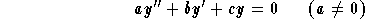where a, b and c are constant. This type of equation is very useful in many applied problems (physics, electrical engineering, etc..). Let us summarize the steps to follow in order to find the general solution:

(1)
Write down the characteristic equation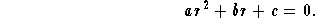This is a quadratic equation. Let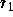and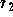be its rootswe have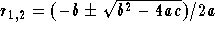;

(2)
Ifandare distinct real numbers (this happens if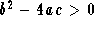), then the general solution is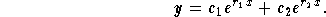(3)
If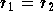(which happens if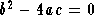), then the general solution is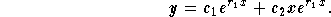(4)
Ifandare complex numbers (which happens if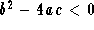), then the general solution is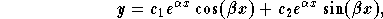where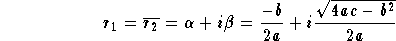,

that is,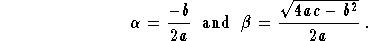Example: Find the solution to the IVP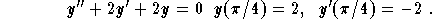Solution: Let us follow the steps:

1
Characteristic equation and its roots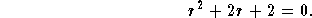Since 4-8 = -4<0, we have complex roots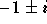. Therefore,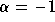and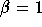;

2
General solution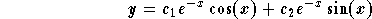;

3
In order to find the particular solution we use the initial conditions to determine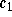and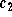. First, we have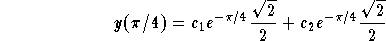.

Since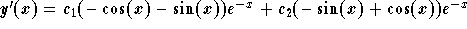, we get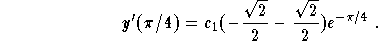From these two equations we get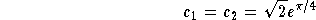,

which implies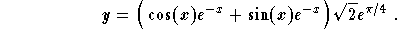[Differential Equations] [First Order D.E.] [Second Order D.E.]
[Geometry] [Algebra] [Trigonometry ]
[Calculus] [Complex Variables] [Matrix Algebra]S.O.S MATHematics home page

Do you need more help? Please post your question on our S.O.S. Mathematics CyberBoard.Author: Mohamed Amine Khamsi